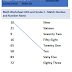# 38 Math Worksheets Printable PDF for Class 1

Sale!

19.00

38 Math Worksheets Printable PDF for Class 1

Kids love to learn Maths from worksheets.

Buy this worksheets bundle and download all worksheets in a bundle (.zip) in one click.

Before buying please review list of worksheet topics and worksheets ( refer Product Description below ).

Once satisfied, buy these worksheets for your kids. Happy Learning !

## Description

38 Math Worksheets Printable PDF for Class 1

•Multiplication
•Match Number and Number Name
•Time – Later or Earlier
•Clock
•Greatest and Least Number
•Multiplication table of 8 and 9
•Multiplication table of 6 and 7
•Multiplication table of 4 and 5
•Multiplication table of 2 and 3
•Subtraction Two consequent borrow
•Subtraction Borrow
•Introduction to Multiplication – Doubling a number
•ADDITION AND SUBTRACTION – 10 MORE or 10 LESS – Number up to 100
•Write missing number in addition – Number up to 100
•Clock Time
•Write missing number in addition by Crossing and Counting Objects – Number
•Days of Week
•Shapes – Sides and Corners
•Number Addition and Writing Number in Words
•Addition of numbers with carry
•Logical Reasoning Worksheet – Grade 1, Grade 2 and Grade 3
•Find the Numbers Using Subtraction or Addition – Worksheet 1
•Fill the missing numbers –Up to 100 – Worksheet 1
•Understanding Tens and Ones using blocks and cubes – Worksheet 1
•Arrange the number from biggest to smallest – Numbers Up to 100 – Worksheet 1
•Arrange the number from smallest to biggest – Numbers Up to 100 – Worksheet 1
•Place value two digit number using Abacus – Numbers Up to 100 – Worksheet 1
•Write Number by reading number names – Numbers Up to 100 – Worksheet 1
•Write Number Names – Numbers Up to 100 – Worksheet 1
•Fill the number series – Numbers Up to 200 – Worksheet 1
•Greater than ( > ), Less than ( < ) and Equal to ( = ) – Numbers Up to 100 – Worksh
•Before, After and Between Numbers Up to 100 – Worksheet 1
•Subtraction of Tens
•Addition of Tens
•Subtraction of Tens
•Addition of Tens

Categories: